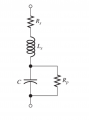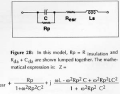# Dissipation Factor of a Capacitor

#### BlackMelon

Joined Mar 19, 2015
147
Hi!

I am trying to use the dissipation factor in a capacitor's datasheet to calculate its series resistance, series inductance, and leakage resistance. For the full model of a capacitor below, should the dissipation factor be calculated as:
Dissipation Factor =(P1+P2)/(Q1+Q2), where P1 and P2 are the average powers of Rs and Rp. Q1 and Q2 are reactive power of Ls and C?BlackMelon

#### BitsNBytes

Joined Mar 22, 2021
20
Hi!

I am trying to use the dissipation factor in a capacitor's datasheet to calculate its series resistance, series inductance, and leakage resistance. For the full model of a capacitor below, should the dissipation factor be calculated as:
Dissipation Factor =(P1+P2)/(Q1+Q2), where P1 and P2 are the average powers of Rs and Rp. Q1 and Q2 are reactive power of Ls and C?
View attachment 285267

BlackMelon
Supposedly:#### Janis59

Joined Aug 21, 2017
1,576
May post a short citation of article:

II. short bits of theory (primer) 

The model of a lossy capacitor uses the term dissipation factor (DF), where DF=ESR/Xc=tan(δ); δ is the loss angle. (formula 1).

Thus the equivalent serial resistance ESR=DF*Xc= =DF/(2*π*f*C) (formula 2),

Thermal outcome flux is P(therm)=i^2*ESR (formula 3).

Sometimes the capacitor quality factor Q is handier in use, where Q=1/DF (formula 4).

For the resonance tank Q(tank)=1/(1/Q{of C}+1/Q{of L}) (formula 5), so deduct that the effort to raise the capacitor Q over the coil Q is rather aimless. As the coil may try to get Q near 1000 and more than 100 is a simple task, then Q of the capacitor may be wished to be in the same range.

Yet for electrotechnical applications often are used the PF (power factor) where PF=cosφ; φ is the phase angle and φ=90º-δ, therefore for a small angle of δ<<10º may be written an approximation tanδ=sinδ=cosφ creating the bold statement of more general approximation: DF=PF (formula 6).

Heating (power flux) at a given circulating reactive power is P(th)=P(circ)*PF (formula 7).

For the capacitor case it looks P(th)=P(circ)*DF (formula 8).

The DF is mostly given in catalogs at one fixed frequency, thus for any other effective work frequency of choice this pre-given DF(0) in that region where DF is diminishing by frequency, can be recalculated.

DF(eff)=DF(0)*sqrt[f(eff)/f(0)] (formula 9).

Using this formula must be considered that at frequencies far below insulator material molecular or atomic resonances, the response curve is typically flat. In the region just below that resonance (or multiple resonances) is according to the formula, but the region over the resonance capacitor may revert and become degraded “be more coil or resistor like a capacitor”. From that frequency region designers try to avoid.